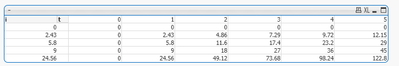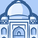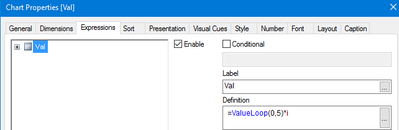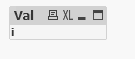Announcements
Product Release Webinar: Qlik Insider airing December 6! REGISTER TODAY!
cancel
Showing results for
Did you mean:Creator

## Calculation within Tables

Hi all,

I have the following table (Input) as an example:

 a b c d e f h i 1 6 0 1,1 2,1 0 0 9 2 0 0 2,3 3,2 1 29 5,8 3 0 0 3,4 4,3 0 1,25 0 4 0 0 4,5 5,4 0 3,33 0 5 0 0 5,6 6,5 1 0 2,43 6 0 1 6,7 7,6 0 0 24,56 0 0 0 0 0 0 0 0

Desired intermediate output (Table):

respecting those formula:

j=m*c (m: entered value from the user with an input box)

k=n*d (n: entered value from the user with an input box)

l=e

vMAX=MAX(j,k,l)

respecting this formula, desired first output looks like:

P[i][t]=(vMax*t-x)(1-f)+(((t-(1/vMax))/o)+1)*f // x=0 if t=o else x=0,001

 i/t 1 2 3 4 5 6 ... o 1 P 2 3 4 P[i][t] 5 6 P[o]

respecting to this formula, second desired output looks like:

 q SUM(h*Transpose(P[t]) SUM(h*Transpose(P[t])

Labels (4)

• ### tables if columns

1 Solution

Accepted SolutionsMaster

this is what i did

13 RepliesMaster

some missing info:  where do you get fields t, x, and o?Creator
Author

o= given from the user with an input box

t increments for example within a for loop from the value 1 to o. (For t=1 to o)

t=o (not zero) -> x=0 (zero), otherweise x=0,005Master

create a pivot table and use values loops as dimensions in place of i and t in your expression:

t:  =valueloop(0,\$(o))  - this will generate fields in your table

the problem though with your expression is that you are not aggregating your fields:

P[i][t]=(vMax*t-x)(1-f)+(((t-(1/vMax))/o)+1)*f

even vMax is not aggregated and since you have multiple values (multiple rows) this expression will be null.  unless you either add all your fields or the user selects one row from your table containing fields a..iCreator
Author

Since my expression does not need aggr. functions, I need to group by a created field with the valueloop to have different values.

The generation of the columns is manually doable, but how can I generate multiple columns with a loop?

How can this code look like?Master

your expression P[i][t] - does the i refer to the field?  and t refers to a generated number from 0 to o?

value loop will generate the individual values you need for t.  there really is no loop in the traditional sense of loops (like for next or do while)

in your expression, you need to generate a matrix of values (i, t).  where i is the field and t is from 0 to o (entered by user)

in your pivot chart, create 2 dimensions:

i -> this is the field
valueloop(0,\$(o)) -> this is t

in your expression, replace all t fields with valueloop(0,\$(o))

you dont need to group by i and t value loop, also encapsulate your vMax variable with \$() - \$(vMax)
vMax should not be immediately evaluated the expression should be
rangemax(m*c, n*d, e)
this way you evaluate all values for c, d, and eMaster

here is a sample pivot:using your data i and valueloop(0,\$(o)), where o =5
in the expression i used
=ValueLoop(0,\$(o)) * i

just to prove the use of valueloop
you can then expand on thatContributor

@AmCh wrote:

Hi all,

I have the following table (Input) as an example:

 a b c d e f h i 1 6 0 1,1 2,1 0 0 9 2 0 0 2,3 3,2 1 29 5,8 3 0 0 3,4 4,3 0 1,25 0 4 0 0 4,5 5,4 0 3,33 0 5 0 0 5,6 6,5 1 0 2,43 6 0 1 6,7 7,6 0 0 24,56 0 0 0 0 0 0 0 0

Desired intermediate output (Table):

respecting those formula:

j=m*c (m: entered value from the user with an input box)

k=n*d (n: entered value from the user with an input box)

l=e

vMAX=MAX(j,k,l)

respecting this formula, desired first output looks like:

P[i][t]=(vMax*t-x)(1-f)+(((t-(1/vMax))/o)+1)*f // x=0 if t=o else x=0,001

 i/t 1 2 3 4 5 6 ... o 1 P 2 3 4 P[i][t] 5 6 P[o]

respecting to this formula, second desired output looks like:

 q SUM(h*Transpose(P[t]) SUM(h*Transpose(P[t])

Table Calculations and Calculated Fields are similar in the sense that they both use functions to compute the results. The difference is how and where the computing takes place, where the result is saved and if it can be reused in more worksheets.Creator
Author

I tried it, but I didn't get it:

Dimensions: i and t as ValueLoop from 1 to 5and the expression as your example:

t*iI got this 'table':Where is the problem here?